# Praxis I Math: Equations and Inequalities Chapter Exam

Exam Instructions:

Choose your answers to the questions and click 'Next' to see the next set of questions. You can skip questions if you would like and come back to them later with the yellow "Go To First Skipped Question" button. When you have completed the practice exam, a green submit button will appear. Click it to see your results. Good luck!

### Page 1

#### Question 1 1. For these questions, I strongly recommend using either the CLEP calculator (found online) or one you use in class. Find x.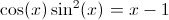#### Question 5 5. For these questions, I strongly recommend using either the CLEP calculator (found online) or one you use in class. Where is the maximum value of f(x) between 0 and pi?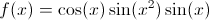### Page 2

#### Question 9 9. This graph corresponds to which inequality?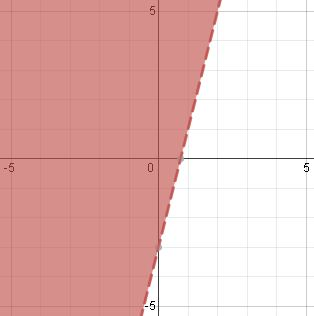### Page 3

#### Question 14 14. What are the coordinates of this point?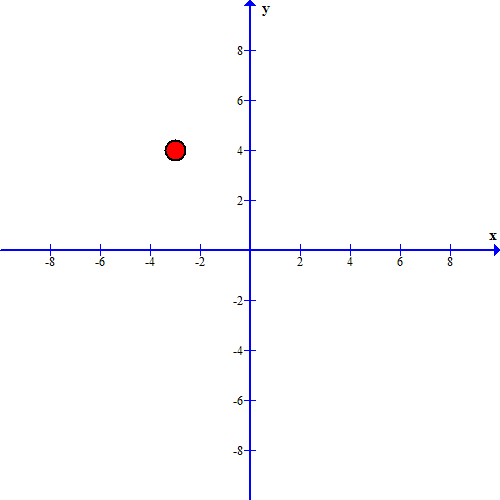#### Question 15 15. If the graph below has the equation Ax + By = -4, what must be true about A and B?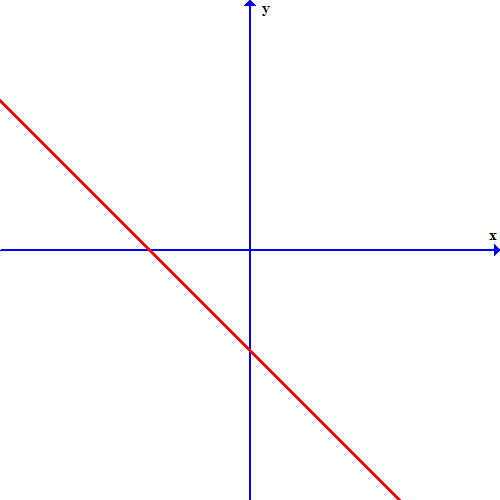### Page 4

#### Question 18 18. For these questions, I strongly recommend using either the CLEP calculator (found online) or one you use in class. What is the root of f(x)? (where f(x) is equal to zero)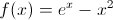### Page 5

#### Question 23 23. This graph corresponds to which inequality?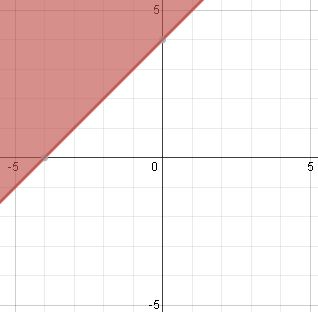### Page 6

#### Question 27 27. What is the equation of the graph below?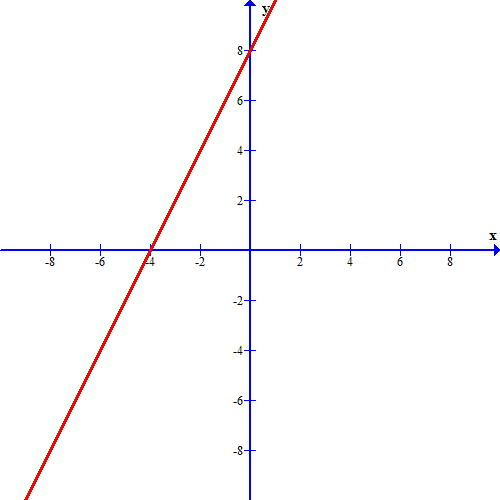#### Question 28 28. Which one of the following equations could work for the following graph?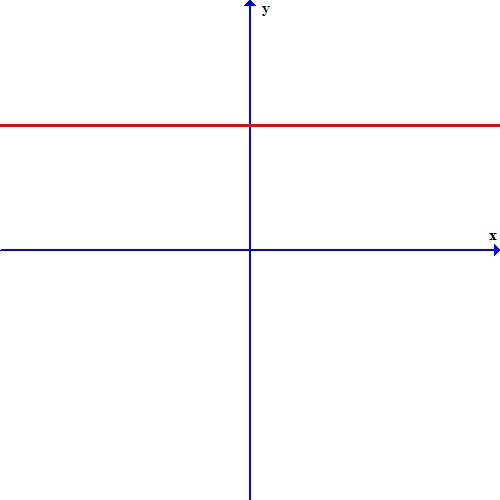#### Question 30 30. If the graph below has the equation Ax+By=C, which statement MUST be true?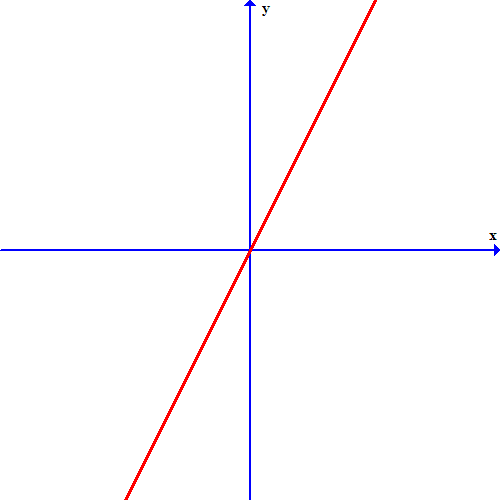#### Praxis I Math: Equations and Inequalities Chapter Exam Instructions

Choose your answers to the questions and click 'Next' to see the next set of questions. You can skip questions if you would like and come back to them later with the yellow "Go To First Skipped Question" button. When you have completed the practice exam, a green submit button will appear. Click it to see your results. Good luck!

Support